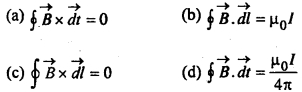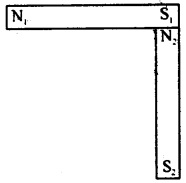# CBSEtips.in

## Thursday, 28 January 2021

### CBSE Class 12 Physics - MCQ and Online Tests - Unit 4 - Moving Charges and Magnetism

#### CBSE Class 12 Physics – MCQ and Online Tests – Unit 4 – Moving Charges and Magnetism

Every year CBSE conducts board exams for 12th standard. These exams are very competitive to all the students. So our website provides online tests for all the 12th subjects. These tests are also very effective and useful for those who preparing for competitive exams like NEET, JEE, CA etc. It can boost their preparation level and confidence level by attempting these chapter wise online tests.

These online tests are based on latest CBSE Class 12 syllabus. While attempting these our students can identify the weak lessons and continuously practice those lessons for attaining high marks. It also helps to revise the NCERT textbooks thoroughly.

#### CBSE Class 12 Physics – MCQ and Online Tests – Unit 4 – Moving Charges and Magnetism

Question 1.
A charged particle of mass m and charge q travels on a circular path of radius r i.e., perpendicular to the magnetic field B. The time taken by particle to complete on revolution is :
(a) $$\frac{2πqB}{m}$$
(b) $$\frac{2πm}{qB}$$
(c) $$\frac{2πmq}{B}$$
(d) $$\frac{2πq^2B}{m}$$

Answer: (b) $$\frac{2πm}{qB}$$

Question 2.
When we double the radius of a coil keeping the current through it unchanged, what happens to the magnetic field directed along its axis at far off points?
(a) halved
(b) doubled
(d) remains unchanged

Question 3.
The sensitivity of a tangent galvanometer can be increased by increasing:
(a) the radius of the coil
(b) the external magnetic field
(c) the number of turns of the coil
(d) all the above

Answer: (b) the external magnetic field

Question 4.
A current carring coil is placed in a uniform magnetic field. If the coil turns through an angle θ, then the torque is directly proportional to:
(a) sin θ
(b) cos θ
(c) cot θ
(d) tan θ

Question 5.
Which of the following is not a unit of magnetic induction?
(a) gauss
(b) tesla
(c) oersted
(d) weber/metre²

Question 6.
The strength of the magnetic field around an infinite current carrying conductor is :
(a) same everywhere
(b) inversely proportional to the distance
(c) directly proportional to the distance
(d) None of these

Answer: (b) inversely proportional to the distance

Question 7.
A current carrying power line carries current from west to east. Then the direction of the magnetic field 2 m above it is :
(a) west to east
(b) south to north
(c) north to south
(d) None of these

Question 8.
According to Ampere’s Circuital lawQuestion 9.
The force between two parallel current carrying conductors is F. If the current in each conductor is doubled, then the force between them becomes :
(a) 4F
(b) 2F
(c) F
(d) $$\frac{F}{4}$$

Question 10.
What happens to the magnetic field at the centre of a circular current carrying coil if we double the radius of the coil keeping the current unchanged?
(a) halved
(b) doubled
(d) remains unchanged

Question 11.
How much force will be experienced by a moving charge in a magnetic field? The symbols have their usual meanings.
(a) $$\vec{F}$$ = q($$\vec{v}$$.$$\vec{B}$$)
(b) $$\vec{F}$$ = $$\frac{q}{(\vec{v}.\vec{B})}$$
(c) $$\vec{F}$$ = $$\frac{q}{2}$$$$(\vec{v}×\vec{B}$$)
(d) $$\vec{F}$$ = q($$\vec{v}$$×$$\vec{B}$$)

Answer: (d) $$\vec{F}$$ = q($$\vec{v}$$×$$\vec{B}$$)

Question 12.
The magnetic field produced by an / meter long straight (x cry thin) current (I) carry ing conductor at any point on itself is :
(a) zero
(b) infinite
(c) $$\frac{µ_0l}{4πl}$$
(d) $$\frac{µ_0l}{2πl}$$

Question 13.
The current sensitibility of a moving coil galanometer increases with decrease in:
(a) magnetic field
(b) area of a coil
(c) number of turns
(d) None of these

Question 14.
A charge + q is sent through a magnetic field. The force acting on it is maximum w hen the angle between the direction of motion of the charged particle and the magnetic field :
(a) 0°
(b) 45°
(c) 90°
(d) 180°

Question 15.
A charge q moves in a region, where electric field E and magnetic field B both exist, then force on it is :
(a) $$\vec{F}$$ = q($$\vec{v}$$×$$\vec{B}$$)
(b) $$\vec{F}$$ = q{$$\vec{E}$$×($$\vec{v}$$ × $$\vec{B}$$)}
(c) $$\vec{F}$$ = q($$\vec{E}$$ + ($$\vec{B}$$ × $$\vec{v}$$)
(d) $$\vec{F}$$ = q($$\vec{B}$$ + ($$\vec{E}$$ ×$$\vec{v}$$)

Answer: (b) $$\vec{F}$$ = q{$$\vec{E}$$×($$\vec{v}$$ × $$\vec{B}$$)}

Question 16.
An electron having mass ‘m’ and Kinetic energy E enters in uniform magnetic field B perpendicular, then its frequency will be:
(a) $$\frac{eE}{qmB}$$
(b) $$\frac{2πm}{eB}$$
(c) $$\frac{eB}{2πm}$$
(d) $$\frac{2m}{eBE}$$

Answer: (c) $$\frac{eB}{2πm}$$

Question 17.
A wire of length 2 metre carries a current 1 ampere, is bent to form a circle. The magnetic moment of the coil is :
(a) 2π
(b) π/2
(c) π/4
(d) 1/π

Question 18.
The magnetic field of a given length of a ware for single turn coil at its centre is B. Then.its value for two turns of coil will be :
(a) B/4
(b) B/2
(c) 4B
(d) 2B

Question 19.
According to Gauss’s theorem in magnetism, surface integral of magnetic field intensity over a surface (closed or open) is always:
(a) -1
(b) 1
(c) 0
(d) infinity

Question 20.
When charged particle enters-a uniform magnetic field, its K.E.:
(a) remains constant
(b) increases
(c) decreases
(d) becomes zero

Question 21.
To convert galvanometer into voltmeter one should connect :
(a) high resistance in series with galvanometer
(b) low resistance in series with galvanometer
(c) high resistance in parallel with galvanometer
(d) low resistance in parallel with galvanometer

Answer: (a) high resistance in series with galvanometer

Question 22.
Isoclinic lines are the lines joining places with :
(a) equal dip
(b) equal declination
(cj equal dip and declination
(d) None of these

Question 23.
Two identical bar magnets each of dipole moment p and length I are perpendicular to each other as shown in Fig. The dipole moment of the combination is:(a) √2 p
(b) $$\frac{p}{√2}$$
(c) P
(d) 2p

Question 24.
The SI unit of magnetic dipole moment is’
(a) Ampere
(b) Ampere metre²
(c) Tesla
(d) None of these

Question 25.
Earth’s magnetism was discovered by:
(a) Gauss
(b) Oersted
(c) Ampere
(d) Gilbert

Question 26.
The force $$\vec{F}$$ experienced by a particle of charge q moving with velocity $$\vec{v}$$ in a magnetic field $$\vec{B}$$ is given by,
(a) $$\vec{F}$$ = q($$\vec{F}$$ × $$\vec{B}$$)
(b) $$\vec{F}$$ = q($$\vec{B}$$ × ($$\vec{b}$$)
(c) $$\vec{F}$$ = q($$\vec{v}$$ × ($$\vec{B}$$)
(d) $$\vec{F}$$ = q($$\vec{v}$$ × $$\vec{B}$$)

Answer: (a) $$\vec{F}$$ = q($$\vec{F}$$ × $$\vec{B}$$)

Question 27.
Tesla is a unit of:
(a) electric flux
(b) magnetic flux
(c) magnetic field
(d) electric field

Question 28.
The permeability of a paramagnetic substance is:
(a) very large
(b) small but more than unity
(c) less than unity
(d) negative

Answer: (b) small but more than unity

Question 29.
What is the angle of dip at the magnetic poles ?
(a) 30°
(b) 0°
(c) 45°
(d) None of these

Question 30.
10 eV electron is circulating in a plane at right angle to a uniform field of magnetic induction 10-1 Wb/m² (1G). The orbital radius of electron is:
(a) 12 cm
(b) 16 cm
(c) 11 cm
(d) 18 cm

Question 31.
Which of the following shows that the earth behaves as a magnet?
(a) Repulsion between like poles .
(b) Attraction between unlike poles
(c) Null points in the magnetic field of a bar magnet
(d) No existence of isolated magnetic poles

Answer: (c) Null points in the magnetic field of a bar magnet

Question 32.
A cyclotron can be used to produce high energy:
(a) neutrons
(b) deutrons
(c) β particles
(d) α partifcles

Question 33.
The radius of the trajectory of a charged particle in a uniform magnetic field is proportional to the:
(a) charge on the particle
(b) energy of the particle
(c) momentum of the particle
(d) all the above

Answer: (c) momentum of the particle

Question 34.
The most suitable metal for making permanent magnets is :
(a) iron
(b) steel
(c) copper
(d) aluminium

Question 35.
Circular loop of radius 0.0157 m carries a current 2 A. The magnetic field at the centre of the loop is :
(a) 1.57 × 10-3Wb/m²
(b) 8.0 × 10-5 Wb/m²
(c) 2.0 × 10-3 Wb/m²
(d) 3.l4 × 10-1 Wb/m²

Answer: (b) 8.0 × 10-5 Wb/m²

Share: